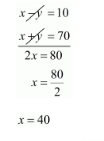# Point A and B are 70 km. a part on a highway.

Question:

Point A and B are 70 km. a part on a highway. A car starts from A and another car starts from B simultaneously. If they travel in the same direction, they meet in 7 hours, but if the travel towards each other, the meet in one hour. Find the speed of the two cars.

Solution:

We have to find the speed of car

Let $X$ and $Y$ be two cars starting from points $A$ and $B$ respectively. Let the speed of car $X$ be $x \mathrm{~km} / \mathrm{hr}$ and that of car $Y$ be $y \mathrm{~km} / \mathrm{hr}$.

Case I: When two cars move in the same directions:

Suppose two cars meet at point $Q$, Then,

Distance travelled by car $X=A Q$

Distance travelled by car $Y=B Q$

It is given that two cars meet in 7 hours.

Therefore, Distance travelled by car $X$ in 7 hours $=7 x \mathrm{~km}$

$A Q=7 x$

Distance traveled by car $y$ in 7 hours $=7 y \mathrm{~km}$

$B Q=7 Y$

Clearly $A Q-B Q=A B$

$7 x-7 y=70$

Dividing both sides by common factor 7 we get,

$x-y=10 \cdots(i)$

Case II : When two cars move in opposite direction

Suppose two cars meet at point. Then,

Distance travelled by car $X=A P$,

Distance travelled by car $Y=B P$.

In this case, two cars meet in 1 hour

Therefore Distance travelled by car $X$ in 1 hour $=1 x \mathrm{~km}$

$A P=1 x$

Distance travelled by car $Y$ in 1 hour $=1 y \mathrm{~km}$

$B P=1 y$

From the above clearly,

$A P+B P=A B$

$A P+B P=A B$

$x+y=70 \ldots$ (ii)

By solving equation (i) and (ii), we getSubstituting $x=40$ in equation (ii) we get

$x+y=70$

$40+y=70$

$y=70-40$

$y=30$

Hence, the speed of car starting from point $\mathrm{A}$ is $40 \mathrm{~km} / \mathrm{hr}$.

The speed of car starting from point $B$ is $30 \mathrm{~km} / \mathrm{hr}$.